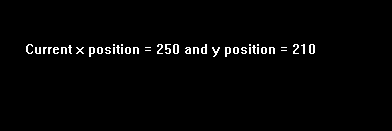# moverel() function in C

The header file graphics.h contains moverel() function which moves a point from the current position(x_pos1, y_pos1) to a point that is at a relative distance (x, y) from the Current Position and then advances the Current Position by (x, y).
Note : getx and gety can be used to find the current position.

Syntax :

```moverel(int x, int y);

The end position is calculated as :
x_pos2 = x_pos1 + x;
y_pos2 = y_pos1 + y;
```

Examples :

```Input : x_pos1 = 0, y_pos1 =0, x = 200, y = 100
Output :Input : x_pos1 = 100, y_pos1 = 150, x = 150, y = 60
Output :```

CASE 1 : When the current position is at origin i.e, (0, 0)

Below is the implementation of moverel() function in C:

 `// C Implementation for moverel() ` `#include ` `#include ` ` `  `// driver code ` `int` `main() ` `{ ` `    ``// gm is Graphics mode which is ` `    ``// a computer display mode that ` `    ``// generates image using pixels. ` `    ``// DETECT is a macro defined in ` `    ``// "graphics.h" header file ` `    ``int` `gd = DETECT, gm; ` `    ``char` `arr; ` ` `  `    ``// initgraph initializes the ` `    ``// graphics system by loading a ` `    ``// graphics driver from disk ` `    ``initgraph(&gd, &gm, ``""``); ` ` `  `    ``// moverel function ` `    ``moverel(200, 100); ` ` `  `    ``// sprintf stands for “String print”. ` `    ``// Instead of printing on console, ` `    ``// it store output on char buffer ` `    ``// which are specified in sprintf ` `    ``sprintf``(arr, ``"Current x position = %d and "` `             ``"y position = %d"``,getx(), gety()); ` ` `  `    ``// outtext function displays ` `    ``// text at current position. ` `    ``outtextxy(100, 150, arr); ` ` `  `    ``getch(); ` ` `  `    ``// closegraph function closes the ` `    ``// graphics mode and deallocates ` `    ``// all memory allocated by ` `    ``// graphics system . ` `    ``closegraph(); ` ` `  `    ``return` `0; ` `} `

Output :

``````

CASE 2 : When the current position is other than origin(100, 150).

Below is the implementation of moverel() function in C:

 `// C Implementation for moverel() ` `#include ` `#include ` ` `  `// driver code ` `int` `main() ` `{ ` `    ``// gm is Graphics mode which is ` `    ``// a computer display mode that ` `    ``// generates image using pixels. ` `    ``// DETECT is a macro defined in ` `    ``// "graphics.h" header file ` `    ``int` `gd = DETECT, gm; ` `    ``char` `arr; ` ` `  `    ``// initgraph initializes the ` `    ``// graphics system by loading a ` `    ``// graphics driver from disk ` `    ``initgraph(&gd, &gm, ``""``); ` ` `  `    ``// moveto function to change ` `    ``// initial position ` `    ``moveto(100, 150); ` ` `  `    ``// moverel function ` `    ``moverel(150, 60); ` ` `  `    ``// sprintf stands for “String print”. ` `    ``// Instead of printing on console, ` `    ``// it store output on char buffer ` `    ``// which are specified in sprintf ` `    ``sprintf``(arr, ``"Current x position = %d and "` `             ``"y position = %d"``,getx(), gety()); ` ` `  `    ``// outtext function displays ` `    ``// text at current position. ` `    ``outtextxy(100, 150, arr); ` ` `  `    ``getch(); ` ` `  `    ``// closegraph function closes the ` `    ``// graphics mode and deallocates ` `    ``// all memory allocated by ` `    ``// graphics system . ` `    ``closegraph(); ` ` `  `    ``return` `0; ` `} `

Output :

``````

Attention reader! Don’t stop learning now. Get hold of all the important DSA concepts with the DSA Self Paced Course at a student-friendly price and become industry ready.

My Personal Notes arrow_drop_upCheck out this Author's contributed articles.

If you like GeeksforGeeks and would like to contribute, you can also write an article using contribute.geeksforgeeks.org or mail your article to contribute@geeksforgeeks.org. See your article appearing on the GeeksforGeeks main page and help other Geeks.

Please Improve this article if you find anything incorrect by clicking on the "Improve Article" button below.

Improved By : Akanksha_Rai

Article Tags :
Practice Tags :

Be the First to upvote.

Please write to us at contribute@geeksforgeeks.org to report any issue with the above content.